Courses

# Test: Pipelining- 2

## 13 Questions MCQ Test GATE Computer Science Engineering(CSE) 2022 Mock Test Series | Test: Pipelining- 2

Description
This mock test of Test: Pipelining- 2 for Computer Science Engineering (CSE) helps you for every Computer Science Engineering (CSE) entrance exam. This contains 13 Multiple Choice Questions for Computer Science Engineering (CSE) Test: Pipelining- 2 (mcq) to study with solutions a complete question bank. The solved questions answers in this Test: Pipelining- 2 quiz give you a good mix of easy questions and tough questions. Computer Science Engineering (CSE) students definitely take this Test: Pipelining- 2 exercise for a better result in the exam. You can find other Test: Pipelining- 2 extra questions, long questions & short questions for Computer Science Engineering (CSE) on EduRev as well by searching above.
QUESTION: 1

### A 5 stage pipeline with the stages taking 1, 1, 3, 1, 1 units of time has a throughput of

Solution:

First output will come after 5 time units, then after every 3 time units, we will get the output. So throughput 1/3.

QUESTION: 2

### What is the control unit’s function in the CPU?

Solution:

The control unit is a component of a computer’s control processing unit that directs the operations of the processor. Control unit transfer the data between registers of CPU or microprocessor and ALU and decode the instruction for execution (ALU).

QUESTION: 3

### Which of the following statements is false with regard to instruction pipelining?

Solution:

The primary goal of instruction pipelining is to achieve a CPI of 1 but not more than 1.

QUESTION: 4

The performance of a pipelined processor suffers if _______.

Solution:

The performance of a pipelined processor depends upon delays of different stage and its hardware resources also it depends upon consecutive instructions format.

QUESTION: 5

Pipelined operation is interrupted whenever two operations being attempted in parallel need same hardware component. Such a conflict can occur if _______.

Solution:

Pipelined operation is interrupted whenever two operations being attempted in parallel need the same hardware component, such a conflict can occur if the execution phase of an instruction requires access to the main memory, which also contains the next instruction that should be fetched at the same time.

QUESTION: 6

Consider the unpipelined machine with 10 ns clock cycles. It uses four cycles for ALU operations and branches, whereas five cycles for memory operations. Assume that the relative frequencies of there operations are 40%, 20%, 40% respectively. Suppose that due to clock skew and setup, pipelining the machine adds 1 ns overhead to the clock. How much speed up in the instruction execution rate will we gain from a pipeline?

Solution: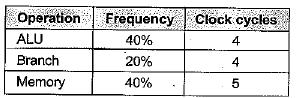Total clock cycle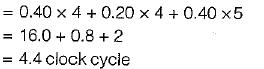Under non pipeline time = 4.4 x (10) = 44
Under pipeline time
= 10 + overhead = 10 + 1 = 11
So, speedup  = 44/11 = 4 times

QUESTION: 7

Using a sequential implementation, it takes a total of 320 ns for each instruction, 300 ns for the combinational logic to complete, and 20 ns to store the result (in a register). This means that a throughput will be about 3.12 millions instructions/second. Assuming you switch to a 3 stage pipeline by splitting the combinational logic into three equal parts and all registers take 20 ns to store results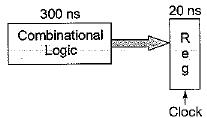How long will it take for a single instruction to execute in the pipelined implementation?

Solution:

Pipelined version will take
3 x 100 + 3 x 20 = 360 ns per instruction

QUESTION: 8

Using a sequential implementation, it takes a total of 320 ns for each instruction, 300 ns for the combinational logic to complete, and 20 ns to store the result (in a register). This means that a throughput will be about 3.12 millions instructions/second. Assuming you switch to a 3 stage pipeline by splitting the combinational logic into three equal parts and all registers take 20 ns to store results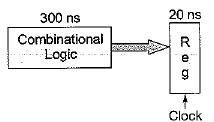By assuming the pipeline never starts, what will the improvement in throughput be?

Solution:

As we calculate, for single instruction pipelined version will take
3 x 100 + 3 x 20 = 360 ns per instructions
∴ Throughput will improve to ⇒ 3 instructions/ 360 ns

QUESTION: 9

A 5 stage pipeline is used to overlap all the instructions except the branch instructions. The target of the branch can’t be fetched till the current instruction is completed. What is throughput of the system if 20% of instructions are branch instructions? Ignore the overhead of buffer register. Each stage is having same amount delay. The pipeline clock is 10 ns. Branch penalty is of 4 cycles.

Solution: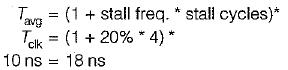∴ Throughput = Number of instructions executed/ sec
18 ns ........... one instruction
109 ns ......... ?
⇒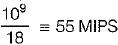QUESTION: 10

A pipelined processor uses a 4-stage instruction pipeline with the following stages: instruction fetch (IF), Instruction decode (ID), Execute (EX) and Write-back (WB). The arithmetic operations as well as the load and store operations are carried out in the EX stage. The sequence of instructions corresponding to the statement X = (S - R * (P+ G))/T is given below. The values of variables P, Q, R, S and T are available in the registers R0, R 1, R2, R3 and R4 respectively, before the execution of the instruction sequence.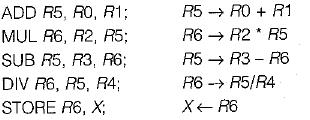Q. The number of Read-After-Write (RAW) dependencies, Write-After-R ead (WAR) dependencies, and Write-After-Write (WAW) dependencies in the sequence of instructions are, respectively,

Solution:

Their are 2 WAW dependencies present between I1 and I3, l2 and I6.
Their are 2 WAR dependencies present between I2 and I5, I3 and I6.
Their are 4 RAW dependencies present between I1 and I2, I2 and I3, I2 and I4, I4 and I5.

QUESTION: 11

A pipelined processor uses a 4-stage instruction pipeline with the following stages: instruction fetch (IF), Instruction decode (ID), Execute (EX) and Write-back (WB). The arithmetic operations as well as the load and store operations are carried out in the EX stage. The sequence of instructions corresponding to the statement X = (S - R * (P+ G))/T is given below. The values of variables P, Q, R, S and T are available in the registers R0, R 1, R2, R3 and R4 respectively, before the execution of the instruction sequence.Q. The IF, ID and WB stages take 1 clock cycle each. The EX stage takes 1 clock cycle each for the ADD, SUB and STORE operations, and 3 clock cycles each for MUL and DIV operations. Operand forwarding from the EX stage to the ID stage is used. The number of clock cycles required to complete the sequence of instructions is

Solution: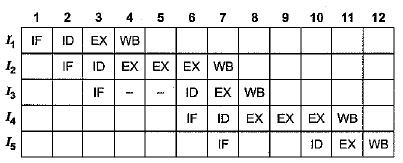Their are 12 clock cycles are required to complete all instruction.

QUESTION: 12

We have two designs D1 and D2 for a synchronous pipeline processor. D1 has 5 pipeline stages with execution times of 3 nsec, 2 nsec, 4 nsec, 2 nsec and 3 nsec while the design D2 has 8 pipeline stages each with 2 nsec execution time. How much time can be saved using design D2 over design D1 for executing 100 instructions?

Solution:

Execution time for Pipeline
= (K + n - 1) x execution _time
Where, k = Number of stages in pipeline
n = Number of instructions execution time
= Max (all stages execution time)
D1 - (5 + 100 - 1 ) x 4 = 416
D2 = (8 + 100 - 1) x 2 = 214
Time saved using: D2 = 416 - 214 = 202

QUESTION: 13

Suppose that an unpipelined processor has a cycle time of 25 ns, and that its datapath is made up of modules with latencies of 2,3,4,7,3,2 and 4 ns (in that order). In pipelining this processor, it is not possible to rearrange the order of the modules (for examples, putting the register read stage before the instruction decode stage) or to divide a module into multiple pipeline stages (for complexity reasons). Given pipeline latches with 1 ns latency:

If the processor is divided into the rewest number of stages that allow is to achieve the minimum latency from part 1, what is the latency of the pipeline?

Solution: